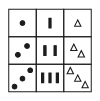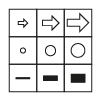# Identify the Missing Symbol to Complete the 4 Box Grid

In this worksheet, students will find the missing symbol to complete a grid of six boxes. It will develop their ability to find and follow patterns in shapes and symbols.Key stage:  KS 2

Curriculum topic:   Spatial and Non-Verbal Reasoning

Curriculum subtopic:   Complete the Grid

Difficulty level:#### Worksheet Overview

It’s time to be a puzzle perfecter!

In these questions, we have been given a grid and we need to work out the missing puzzle piece.

Grids can follow a horizontal pattern which goes across or a vertical pattern which goes up or down.

To find the missing puzzle piece, we first need to think about what the pattern might be.

Here are some example patterns and how they change in the grid:

 Pattern Example Grid Number of shapes increases.Shading gets lighter/darker.Size of the image increases/decreases.The image rotates (turns).The number of sides of the shapes in the image increases.Take a look at this example:Which of the following images would complete this grid?
a)b)c)d)The pattern in this case is horizontal.

In each square of the grid, the number of sides of each shape increases by one each time. So the answer is c!

It’s now your turn to find the missing puzzle piece in grids.

Remember to decide if it is horizontal or vertical when working out what the pattern is. Good luck puzzle champ!